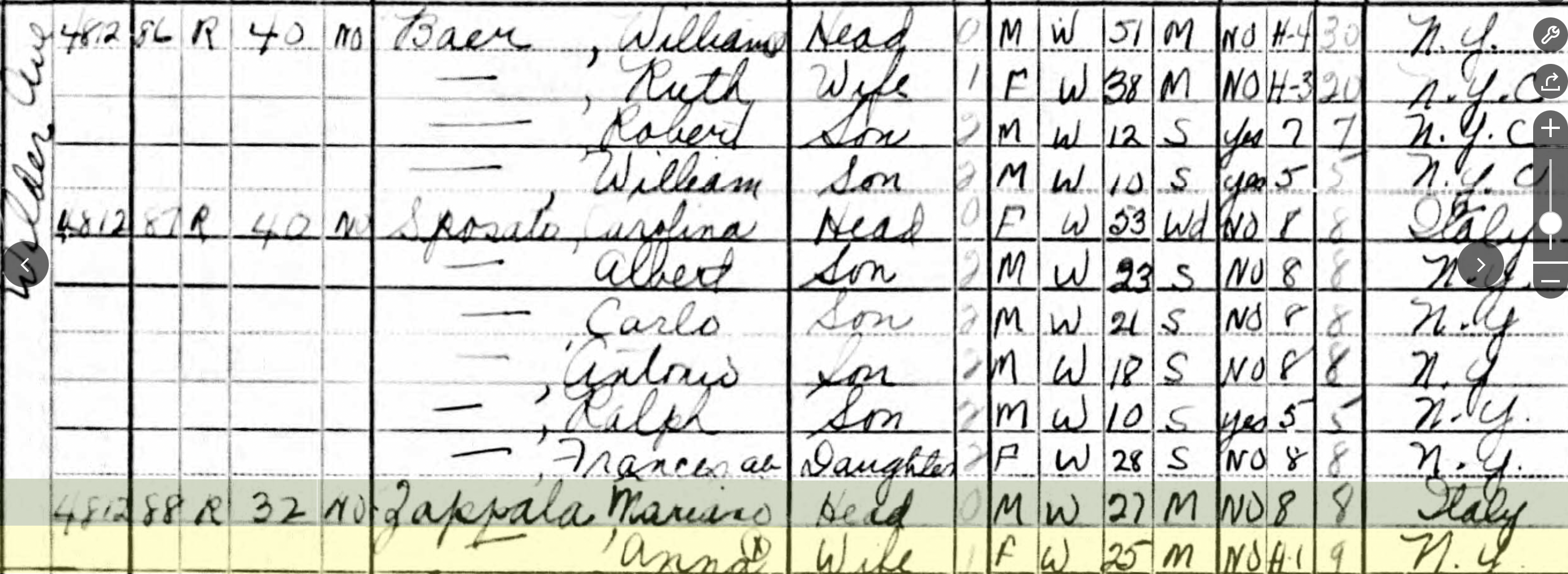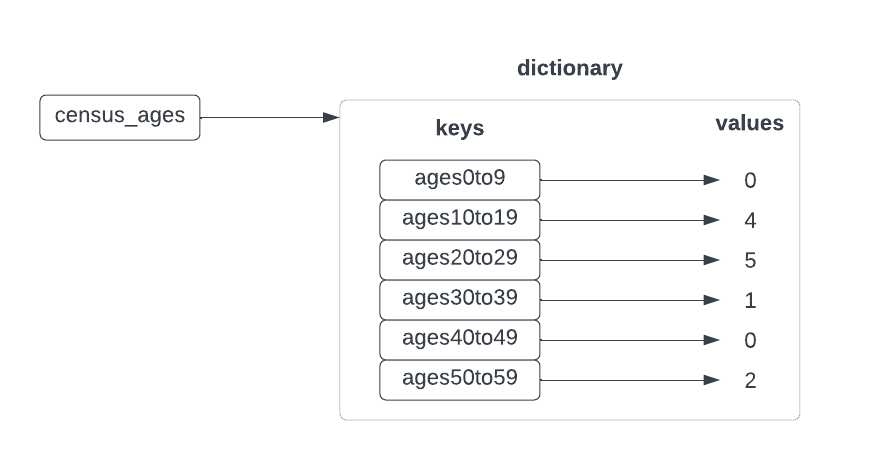Computer Science

# Dictionaries, Part 1

• Let’s imagine you have some census data from 1940:• You get this data in comma-separated value format (CSV)
``````# last, first, relationship, gender, race, age, marital status, ...
Baer,Ruth,wife,F,W,38,M
Baer,Robert,son,M,W,12,S
Baer,William,son,M,W,10,S
Sposato,Albert,son,M,W,23,S
Sposato,Carlo,son,M,W,21,S
Sposato,Antonio,son,M,W,18,S
Sposato,Ralph,son,M,W,10,S
Sposato,Frances,daughter,F,W,28,S
Zappala,Anna,wife,F,W,25,M``````
• You would like to calculate the number of people in the census who are:

• ages 0-9
• ages 10-19
• ages 20-29
• ages 30-39
• ages 40-49
• and so forth

• You could create separate variables for each age range:

``````ages0to9 = 0
ages10to19 = 0
ages20to29 = 0
ages30to39 = 0
ages40to49 = 0``````
• and then use the accumulator pattern, right?• What you want instead is a dictionary:
• maps keys to values## Creating dictionaries

``````# create a blank dictionary
age_count = {}
age_count['age0to9'] = 0
age_count['age10to19'] = 0
age_count['age20to29'] = 0
age_count['age30to39'] = 0
age_count['age40to49'] = 0
age_count['age50to59'] = 0
print(age_count)``````
``    {'age0to9': 0, 'age10to19': 0, 'age20to29': 0, 'age30to39': 0, 'age40to49': 0, 'age50to59': 0}``
``````# shorter version
age_count = {'age0to9': 0, 'age10to19': 0, 'age20to29': 0, 'age30to39': 0, 'age40to49': 0, 'age50to59': 0}
print(age_count)
``````
``    {'age0to9': 0, 'age10to19': 0, 'age20to29': 0, 'age30to39': 0, 'age40to49': 0, 'age50to59': 0}``

## Getting and setting values

``````# get a value
result = age_count['age20to29']
print(result)

# set a value
age_count['age20to29'] = 5
result = age_count['age20to29']
print(result)
``````
``````    0
5``````
• each value acts like any other variable
• it can have only one value
• if you change it, you overwrite the old value
``````result1 = age_count['age20to29']
age_count['age20to29'] = 6
result2 = age_count['age20to29']
print(f"count was {result1} now it is {result2}")``````
``    count was 5 now it is 6``

## Example: Census Count

``def census_age_count(filename):``
• We will do this in class in PyCharm
``````def census_age_count(filename):
"""
Count ages in the census
:param filename: the name of a file with 1940 census data
:return: a dictionary with counts accumulated by age
>>> census_age_count('census.txt')
{'age0to9': 0, 'age10to19': 4, 'age20to29': 5, 'age30to39': 1, 'age40to49': 0, 'age50to59': 2}
"""
age_count = {'age0to9': 0, 'age10to19': 0, 'age20to29': 0, 'age30to39': 0, 'age40to49': 0, 'age50to59': 0}
with open(filename) as file:
for line in file:
last, first, relationship, gender, race, age, marital_status = line.strip().split(',')
age = int(age)
if age < 10:
age_count['age0to9'] += 1
elif age < 20:
age_count['age10to19'] += 1
elif age < 30:
age_count['age20to29'] += 1
elif age < 40:
age_count['age30to39'] += 1
elif age < 50:
age_count['age40to49'] += 1
elif age < 60:
age_count['age50to59'] += 1
return age_count``````

## Checking if a key is in a dictionary

``result = age_count['age90to99']``
``````    ---------------------------------------------------------------------------

KeyError                                  Traceback (most recent call last)

/var/folders/9x/cb134v3d2nb22_rksynbspqm0000gn/T/ipykernel_54731/704241586.py in <module>
----> 1 result = age_count['age90to99']

KeyError: 'age90to99'``````
``````result = 0
if 'age90to99' in age_count:
result = age_count['age90to99']
print(result)``````
``    0``

• given a dictionary `meals` where the key is a ‘breakfast’, ‘lunch’, or ‘dinner’
• the values are foods
• bad start if you didn’t have breakfast or if you had candy for breakfast
``def bad_start(meals):``
``````def bad_start(meals):
if 'breakfast' not in meals:
return True
if meals['breakfast'] == 'candy':
return True
return False

``    True``
``````def bad_start(meals):
if 'breakfast' not in meals or meals['breakfast'] == 'candy':
# if meals['breakfast'] == 'candy' or 'breakfast' not in meals:
return True
return False

return 'breakfast' not in meals or meals['breakfast'] == 'candy'

``````
``    False``

## Example: Enkale

• given a dictionary `meals` where the key is a ‘breakfast’, ‘lunch’, or ‘dinner’
• the values are foods
• if ‘dinner’ has ‘candy’ as a value, change it to kale
• return the dictionary
``def enkale(meals):``
``````def enkale(meals):
if 'dinner' in meals and meals['dinner'] == 'candy':
meals['dinner'] = 'kale'
return meals

enkale({'dinner': 'candy'})``````
``    {'dinner': 'kale'}``

## Example: Is Boring

• given a dictionary `meals` where the key is a ‘breakfast’, ‘lunch’, or ‘dinner’
• the values are foods
• if lunch and dinner are both present and are the same food, return True
``def is_boring(meals):``
``````def is_boring(meals):
if 'lunch' in meals and 'dinner' in meals and meals['lunch'] == meals['dinner']:
return True
return False

def is_boring2(meals):
return 'lunch' in meals and 'dinner' in meals and meals['lunch'] == meals['dinner']

is_boring({'dinner': 'pizza', 'lunch': 'pizza'})
is_boring2({'dinner': 'pizza', 'lunch': 'pizza'})``````
``    True``

## Computing keys

• we have been creating dictionaries like this:
``````meals = {}
meals['breakfast'] = 'candy'
meals['dinner'] = 'pizza'``````
• or this:
``meals = { 'breakfast': 'candy', 'dinner': 'pizza'}``
• but what if we want to compute the keys?
• given a list of words, find a count of all the words starting with each letter
``def count_words_by_starting_letter(words):``
• we will do this in class using PyCharm
``````def count_words_by_starting_letter(words):
"""
count all the words starting with each letter
:param words: a list of words
:return: a dictionary that counts all the words starting with each letter
>>> result = count_words_by_starting_letter(['rock', 'paper', 'scissors', 'stone', 'parchment'])
>>> from pprint import pprint
>>> pprint(result)
>>> {'p': 2, 'r': 1, 's': 2}
"""
starting_letters = {}
for word in words:
letter = word
if letter not in starting_letters:
starting_letters[letter] = 0
starting_letters[letter] += 1
return starting_letters``````

## Let’s revisit census_age_count()

• this was our dictionary:
``age_count = {'age0to9': 0, 'age10to19': 0, 'age20to29': 0, 'age30to39': 0, 'age40to49': 0, 'age50to59': 0}``
• what if we instead want to automatically calculate these?

• we need a function that turns an age into a key:

``def round_to_nearest_10(number):``
• we will write this in class using PyCharm
``````def round_to_nearest_10(number):
"""
Round a number down to the nearest 10
:param number: a number
:return: a number rounded down to the nearest 10
>>> round_to_nearest_10(10)
10
>>> round_to_nearest_10(18)
18
"""
remainder = number % 10
return number - remainder``````
• now we can rewrite `census_age_count()` to calculate our keys instead of having to pre-determine what they are

• we will write this in class using PyCharm

``````def census_age_count2(filename):
"""
Count ages in the census
:param filename: the name of a file with 1940 census data
:return: a dictionary with counts accumulated by age
>>> result = census_age_count2('census.txt')
>>> from pprint import pprint
>>> pprint(result)
{10: 4, 20: 5, 30: 1, 50: 2}
"""
age_count = {}
with open(filename) as file:
for line in file:
last, first, relationship, gender, race, age, marital_status = line.strip().split(',')
age = int(age)
# round by to nearest 10s
age_group = round_to_nearest_10(age)
if age_group not in age_count:
age_count[age_group] = 0
age_count[age_group] += 1
return age_count``````